## 5.3 Properties of these 4 Average and Change Series

### A. The Original or Zeroth term of the 4 Series

#### Some interesting inferences from working backwards

Let us remember the general definitional equation for the Pth Vector Stream.Working backwards from the general equation we come to some interesting inferences about the zeroth term of the Vector Series.

#### A Notational interjection: Zeroth = Origin or Original

The zeroth term of these four series is the Origin of the Series, not the first member. Hence we will refer to the zeroth term of these series as the Origin or Original. Hence the zeroth term of the Vector Series is the Origin Vector or Original Vector.

#### The Original Vector and the Original Directional

Let us look at the 1st and 2nd Vector in terms of the general equation.We also remember that the First Vector is merely the difference between the Data and the Past Average, shown algebraically below.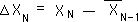Thus the Original Vector is the Raw Data, itself, while the Original Directional is the Decaying Average. The algebra is below.We will repeat this important result. The Decaying Average is the Original Directional and the Raw Data is the Original Vector. The Original Vector represents the difference between existence and non-existence.

#### A Representation of the Directional and the Vector Series, including their Original Term and identity

Below is the representation of the Vector Series, with the identity of the Raw Data Stream and the Original Vector noted by an equality sign, which in this case only includes the first element of the series set, not the whole set. The comma breaks the equality in my notation.Similarly below is the Directional Series with the identity of the origin element and the Average Stream noted.#### A Infinite Set composed of Finite Streams

The first element of the Vector Series is the Data Stream itself. The second element is the stream representing the first level of change based upon the Data Stream. The third element is the stream representing the second level of change based upon the Data Stream. So the Vector Series is a set of Data Streams based upon successive levels of changes. Each of its elements is a Data Stream, but the Vector Series is not. The Vector Series is infinite but is, in theory, complete because, although each of its elements is growing, the Vector Series itself is not growing. It always has the same number of elements.

#### The Origin of the Deviation Series and Scalar Series

We can use the same process to discover the Origin of the Deviation Series and the Scalar Series. First let us start with general definition of the Pth Scalar.#### Working backwards again

Starting with the general definition of a Scalar Stream and working backwards we find that the Origin of the Deviation Set is equal to the Decaying Average and that the Raw Data is the Origin of the Scalar Set.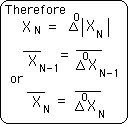#### Parallel Beginnings

But wait a minute; we came to the same conclusion with the Vector Series.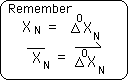The Original Scalar equals the Original Vector, which both equal the Raw Data. Also the Original Deviation is equal to Original Directional which both equal the Average. The point that we're making is that both Change Series, the Scalar and Vector, begin with the same Stream, the Raw Data. Also the Directional Series and Deviational Series begin with the same stream, the Decaying Average Stream.

#### The Representation of the Scalar and Deviation Series

Similarly we represent the Deviation Series and its Change Series, the Scalar Series, with their identities below.### B. Simplification or Losing the Change Series, some Derivations

#### The second Scalar

In terms of calculation, the definitions for the different Scalar levels are prohibitive. The above definitions and derivations are logically derived but are impractical for computation. To find the 6th Scalar for the Nth Data Piece, one needs the 5th Scalar of the Nth Data Piece and the 5th Directional. But to find the 5th Scalar one needs the 4th Scalar and so on. Below is a more practical computational formula based upon the stored Deviations. In the derivations below we eliminate the need to know the Scalars on the prior levels. This simplifies computation of Scalars considerably.#### The Third and Fourth Scalar

Below is the derivation of the simplification of the Third Scalar. The Fourth Scalar follows easily from the Third. The pattern is obvious. The Fourth Scalar for the Data Stream equals the absolute difference between the Present Data Stream and the sum of all the Past Deviations including the Origin.#### A Simplification of the Pth Scalar

The progression to the general level of absolute change is quite straightforward. I'll leave the proof for a more accomplished mathematician. Verbally the theorem goes like this. The Pth Scalar equals the absolute difference between the Present Data and the sum of all the Past Deviations including the Original Deviation.#### The Second Vector

Similarly we would also like to write the higher levels of Vector Streams in terms of the Data and the Directionals only. No more high level Vector streams. They are too difficult to calculate. This also helps us to better understand the underlying structure of these Changes. Below is a derivation, which eliminates the different levels of change from the equations and ends up with the original Data and Directionals only.The above equation defines the Second Vector as the Present Data Stream minus the sum of the Previous Directionals.

#### The Third & Fourth Vectors

The third and fourth levels of change are derived in the same way.#### A Simplification of the Pth Vector Stream

Below is the general equation for the Pth Vector Stream. We will leave the proof for a more thorough mathematician than myself.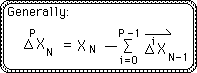#### The Pth Directional

Now that we have established the simplifications for the two change series' we can more easily simplify the general equations for the Deviational and Directional series.Verbally this equation says that the Pth Directional equals the difference between the Present Data Stream and the sum of all the past Directionals of all levels including the origin divided by the decay factor, D, added to the prior directional of the same level as the one sought after. An intriguing and beautiful equation.

#### The Pth Deviation

Using the same reasoning as above we derive a simplification of the Pth Deviation. Notice that in both simplifications that all the elements of the both Change Series drop out, simplifying computation tremendously. The only part of the Change Series is the Raw Data Stream.### C. Casual Proof that the First Deviation is > or = to the First Directional

#### Definitions

These are the definitional equations for the First Deviation and the First Directional.#### Verbal Proof

This is the verbal proof that the first Deviation is greater than or equal to the first Directional.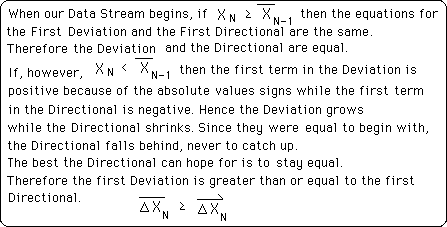### D. Streams vs. Series

#### Streams

Before plowing ahead let us make a firm differentiation between Streams and Series, in the context of our discussion. Below are three Streams. The first, S, is a raw Data Stream. The Data is numbered, ordered. The Stream has no end. There is a specific interval between each piece of Data. The second stream, A, is derived from the first stream. It is the Average Deviation Stream, the stream of average changes or the first level of Deviation. It is ordered, without end, and totally dependent upon the Data Stream for its life. The third stream, D, is also derived from the first stream. It is the First Directional Stream, the stream of first Directionals. It is ordered, without end, and also totally dependent upon the Data Stream for its life.#### Without an End & Finite?

Although a Data Stream is without an end, it is finite. When a Data Stream ends, in some senses it becomes a Static Data Set and ceases to be a Live Stream. It can be treated as a Live Stream but it has now become a Static Set. All Data Streams end eventually, even if they wait until the end of time. The Data Stream of our universe ends with the collapse of the Big Bang into a Black Hole, heavy enough to transform everything recognizable including Time.

#### Series

Below are the two Change Series that we have already introduced, the Vector Series & the Scalar Series. These series are made up of an infinite number of Data Streams. These series are not necessarily ordered, except by necessity of calculation. Otherwise their order has no significance. The Data Streams are ordered because of sequence. Our series are only ordered because of computation.#### Average Series derived from Change Series derived from the Raw Data Stream

Below are the two crucial Average Series, the Directional Series and the Deviation Series. The 2 Change Series are included in, these two Average Series and so will be ignored from now on. While the data from the 2 Change Series are instantaneous, immediate, and gone, kindling for the fire, the Data from the two Average Series are descriptive and predictional of a certain specified point in time. The kindling from the 2 Change Series fuels the fire of the 2 Average Series; the same Raw Data Stream fuels the 2 Change Series. This is the Primary Data Stream. All others are derived from it. If it goes out then all the infinite number of Change Streams are immediately extinguished along with all the infinite number of Average Streams. The Data Stream Series are infinite but finished. There are no unknown elements, although there are an infinite number of them. Each of the elements has unknown parts but the element itself is known. The Data Stream Series is infinite but finished.#### Summary of Differences

The Data Streams are ordered by sequence. The Data Stream Series are ordered by computation. The Data Streams are unfinished and finite, while Data Stream Series are finished and infinite. The Primary Data Stream is the Raw Data itself, upon which all of the other Data Streams and Data Stream Series are based. The elements of the Data Stream Series are an infinite number of Data Streams, while the elements of a Data Stream are a finite number of Data Points.

### E. Dynamical Directionals

#### Definitional Equations for Deviations

Let us review some of our definitions to cement their meaning for the Notebooks that follow. The Decaying Average formula below determines an average, which is sensitive and relevant to the Data Stream. {See Decaying Averages Notebook.} Because of the need to know the boundaries of the Realm of Probability, the First Deviation was calculated by the second formula below. This is the Decaying Average formula applied to the First Scalar. It calculates the average change. Similarly we can apply the same Decaying Average formula to the Second Scalar, which is the Change of Changes. This is represented in the third formula below, the Second Deviation. We can extend this process indefinitely, finding the third, fourth, and Pth Deviations. Below are the Third Deviation and the Pth Deviation.#### Definitional Equations for Directionals

The Directional Series is related to the Derivative Series in Calculus. The Directional Series is related to the rates of changes of a Raw Data Stream while the derivatives of calculus related to the rates of changes of physical objects. There are normally equations for each calculus derivative and then Differential Equations, which relate the equations of the derivatives in an incredibly simplified scheme. No such luck for living creatures. The Data Stream has position, velocity, acceleration, thrust, and impulse, but they are statistically based rather than equation based.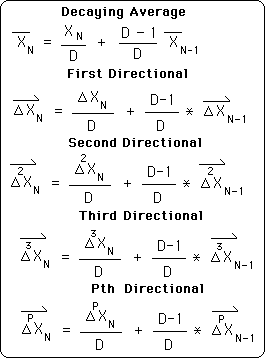The Decaying Average is the root of the Directional Series as well as the Deviational Series. Hence it is included here to begin our Directional Series. It is related to the average position of our Data Stream. Notice that the arrow of the Directionals has replaced the bar of the Deviations, but that equations are parallel.

#### The Directionals parallel with Physics

One last note about the parallels between the physical derivatives of motion and our Data Stream Derivatives, specifically Directionals. The First Directional is related to the velocity of the Data Stream. While the First Differential is similar to the First Deviation, the First Deviation does not take the direction of the change into account. The First Directional measures the average amount of change but takes direction into account. The Deviations measure the wobbliness of the Data Stream while the Directionals measure the rates of changes of our Data Stream, i.e. velocities, accelerations, etc. So the First Directional as is the first Derivative is related to the velocity of the Data Stream. The Second Directional measures the rate of change of the changes. Hence it is related to the acceleration of our Data Stream. The third Directional measures the rate of change of the changes of the changes. Hence it is related to the thrust of the Data Stream. Again it must be stressed that because these are Live Data Streams, unpredictability is a built in feature. Therefore our measures are statistical, descriptive, and predictive, but nowhere near exact like their physical cousins.

#### Directionals only have a meaning in a dynamical system

Remember that Directionals have no meaning in a static Data set. This is the scientists of static Data Sets never discovered them. The only time Directionals have meaning is when they are applied to growing Data Streams. Deviations have their parallel in the Average Deviations or Standard Deviations of Static Data Sets. Also the Decaying Average has its parallel in the Mean Average of the Data Sets. Hence Directionals are the only tool we have developed that is truly unique to our system. Directionals only have a meaning in a dynamical system based upon Data Streams rather than Data Sets.